Chen, Y., and Guo, W. (2017). "Nondestructive evaluation and reliability analysis for determining the mechanical properties of old wood of ancient timber structure," BioRes. 12(2), 2310-2325.

#### Abstract

The objective of this study was to evaluate the mechanical properties of ancient wood of Abies fabri (Mast.) Craib based on the nondestructive tests and reliability analysis. Nondestructive tests including resistograph and stress wave test, and destructive tests were conducted on the wood specimens. Results indicated that there were significant linear correlations between the resistance amplitude (F) and green density (ρ), the dynamic modulus of elasticity (ED) and static modulus of elasticity (MOE), modulus of rupture (MOR), and ultimate compressive strength (UCS) of wood specimens. The cumulative distribution of the predicted MOR and UCS based on the nondestructive tests could be well fitted by the normal distribution according to the χ2 test. Moreover, a reliability analysis program based on the first-order second-moment method was developed. Reliability analysis results showed that the reliability index increased nonlinearly with the increase of the live-to-dead load ratio, and decreased nonlinearly with the increase of the design values for all the simulation load cases. According to the minimum reliability index requirements of the Chinese national standard, it is suggested that the design value of MOR and UCS be 14.0 and 10.7 MPa, respectively.

Nondestructive Evaluation and Reliability Analysis for Determining the Mechanical Properties of Old Wood of Ancient Timber Structure

Yongping Chen* and Wenjing Guo

The objective of this study was to evaluate the mechanical properties of ancient wood of Abies fabri (Mast.) Craib based on the nondestructive tests and reliability analysis. Nondestructive tests including resistograph and stress wave test, and destructive tests were conducted on the wood specimens. Results indicated that there were significant linear correlations between the resistance amplitude (F) and green density (ρ), the dynamic modulus of elasticity (ED) and static modulus of elasticity (MOE), modulus of rupture (MOR), and ultimate compressive strength (UCS) of wood specimens. The cumulative distribution of the predicted MOR and UCS based on the nondestructive tests could be well fitted by the normal distribution according to the χ2 test. Moreover, a reliability analysis program based on the first-order second-moment method was developed. Reliability analysis results showed that the reliability index increased nonlinearly with the increase of the live-to-dead load ratio, and decreased nonlinearly with the increase of the design values for all the simulation load cases. According to the minimum reliability index requirements of the Chinese national standard, it is suggested that the design value of MOR and UCS be 14.0 and 10.7 MPa, respectively.

Keywords: Mechanical properties; Resistograph and stress wave test; Reliability analysis; Ancient timber structure

Contact information: Research Institute of Wood Industry, Chinese Academy of Forestry, Dong xiaofu No 1, Xiangshan Road, Haidian District, Beijing 100091, P. R of China;

* Corresponding author: chenyongping0927@163.com

INTRODUCTION

In many Asian countries, and in particular in China, a large historical heritage of ancient timber structures needs to be preserved. The refurbishment or improvement is carried out on ancient timber structures for the enhancement of itself residual structural capacity of different members. Wood as a natural biomass material is easily subjected to biological degradation because of environmental conditions. Besides, different structural abnormalities such as cracks, splits, shakes, and holes, are often found in the existing wood members. All this can lead to the complexity and variability of the loading condition of wood members. For these reason, reliable tests should be adopted for the evaluation of its mechanical properties (Bertolini et al. 1998; Tampone 2001).

In terms of historical relics, it is known that both local and whole destructive tests are not allowed. These destructive tests can result in the loss of structural integrity. In order to obtain mechanical properties and to evaluate the residual capacity of the existing wood members, nondestructive tests (NDT) can be selected as an effective means (Riggio et al. 2014). Stress wave timing and resistance drilling are the common nondestructive test (NDT) tools, and they can provide qualitative and quantitative support information on the stiffness and strength of existing wood members.

Most of preliminary studies into the NDT have been mainly concerned with the stress wave timing test. The mechanical properties and effective section have been examined by such tests (Wang et al. 2004; Yin et al. 2010; Dackermann et al. 2014). In addition, some research (Rinn et al. 1996; Acuna et al. 2011; Kahl et al. 2009) proposed that a drilling resistance test could provide good prediction for wood density. As reported by Jasieńko et al. (2013), Tannert et al. (2014), and Zhang et al. (2015), the drilling resistance test also could detect the internal defects, cracks, and decay, and determine the size and location. Feio and Machado (2015) and Probett et al. (2013) investigated the relationships between dynamic modulus of elasticity of specimens and modulus of elasticity, bending strength, and ultimate compressive strength parallel to grain by stress wave timing combined with resistance drilling tests. To the author’s knowledge, there has been little research on the prediction of mechanical properties by stress wave timing test combined with drilling resistance test.

Therefore, the objective of this work was to determine the mechanical properties of the ancient wood of Abies fabri (Mast.) Craib by nondestructive test methods (stress wave timing test combined with drilling resistance test) and reliability analysis. The relationships between nondestructive test results and static mechanical tests were examined. The cumulative distribution of the predicted MOR and UCS based on the nondestructive tests were fitted by a normal distribution. Finally, according to the minimum reliability index requirements of the Chinese national standard, the design value of MOR and UCS were determined based on the reliability analysis.

MATERIALS AND METHODS

Preparation of Test Pieces

In the course of a repair process, 10 sample logs were collected from Huguo temple, which has been standing for 1,000 years and is located in Beijing, China. According to the identification by the Chinese Academy of Forestry in Beijing, the wood species of all logs were Faber’s fir (Abies fabri (Mast.) Craib). The moisture content of logs was about 10.0%.

As many as possible small rough specimens (40×40×500 mm) were sawn from the logs, according to the sketch in Fig. 1(a). There were a total of 114 small rough specimens for further testing. Because of the low moisture content, these small rough specimens were directly planed and cut into 20×20×450 mm pieces without the maintenance at a constant temperature and humidity. Considering the limited log diameter and the presence of knots, only 72 pieces were finally selected for both the nondestructive test and mechanical test.

For nondestructive tests, Figs. 1(b) and 1(c) show the stress wave timing test and resistograph test, respectively. An average mean value from two testing points was specified to be the resistance amplitude of each specimen. After nondestructive tests, each wooden specimen was then divided into two parts for static mechanical tests: one 30-mm section for compressive test and one 300-mm section for bending test (Fig. 1(d)). And the flow chart in processing and testing of wood specimen is presented in Fig. 1.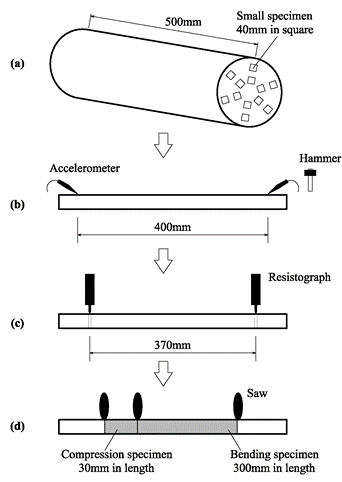Fig. 1. Flow chart in processing and testing of small specimen: (a) Sawing pattern for extracting small specimens; (b) Stress wave velocity measurement; (c) Resistance amplitude measurement; (d) Cutting small specimens for static mechanical test

Nondestructive Tests

For the stress wave timing test, the two spikes of stress wave equipment (Fakopp, Microsecond Timber, Ágfalva, Hungary) were nailed into the xylem at a 45° angle with the log axis. The head of a spike with an accelerometer was hit by a hammer, and the stress wave signal was detected by another accelerometer after wave propagation in the wood specimen. Based on the nondestructive test results and the green density of wood specimen, the dynamic modulus of elasticity, ED, of the specimen could be calculated as,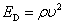(1)

where ED is the dynamic modulus of elasticity of the wood specimen (GPa), ρ is the green density of the wood specimen (g/cm3), and υ is the stress wave velocity propagated in the wood specimen (m/s).

However, it is difficult to determine the green density of the whole or local section of an existing structural member or a standing tree by a traditional testing method, and the destructive tests cannot allow such tests to be carried out. A nondestructive method with the resistograph test technology thus has been developed for the prediction of density (Rinn et al. 1996; Acuna et al. 2011; Kahl et al. 2009). The drill bit with a 1.5 to 3 mm diameter of the resistograph equipment (IML-RESI, PD, Heidelberg, Germany) was driven into the xylem perpendicular to the tangential plane, with a constant feed speed of 2,000 mm/min and needle speed of 5,000 r/min. The average value of resistance amplitude curve from the first peak to the last peak, as shown in Fig. 2, was used to represent the overall density of wood specimen. The higher the average value of resistance amplitude curve was, the higher was the density of the wood specimen. And the dynamic modulus of elasticity of wood specimens (Eq. 1) could be rewritten as,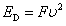(2)

where F is the average value of resistance amplitude of the wood specimen.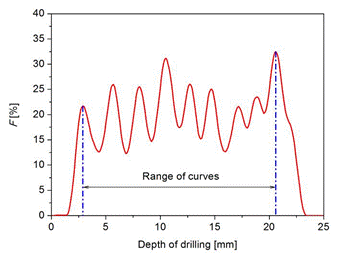Fig. 2. Typical curve of resistance amplitude

Static Mechanical Tests

Every country has its own national standards for the testing methods employed for wood specimens. Based on the Chinese national standards (Table 1), the static bending tests and compressive tests parallel to grain were conducted by using an Instron 5582 Universal Test Machine (Boston, USA), as shown in Fig. 3. A three-point loading method was used for the static MOE test. Likewise, a three-point loading method was applied for the MOR test. Compressive strength was measured parallel to the grain. The static MOE, MOR, and UCS could be expressed as,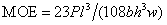(3)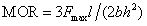(4)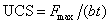(5)

where MOE is the static modulus of elasticity (MPa); MOR is the static modulus of rupture (MPa); UCS is the ultimate compressive strength parallel to the grain (MPa); P is an increment of load (N); l is the distance between two support points (mm); b is the specimen width (mm); h is the specimen height (mm); w is the increment of deformation corresponding to P (mm); Fmax is the maximum load (N); and t is the specimen thickness (mm).

(a)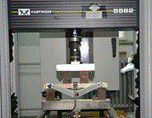(b)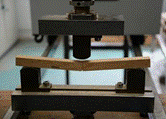(c)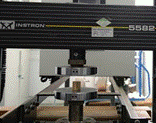Fig. 3. Static mechanical tests: (a) MOE; (b) MOR; (c) UCS

Table 1. Summary of Static Mechanical Test Conditions for Wood SpecimensStatistical Analysis

Statistical analysis was performed using Origin 9.0 software (OriginLab Corporation, Northampton, USA). Coefficient of determination between nondestructive parameters and mechanical properties of wood specimens were also calculated to determine their relationships. The significance level was set to p < 0.05.

RESULTS AND DISCUSSION

Results of Nondestructive and Static Mechanical Tests

Both the nondestructive and static mechanical test results, such as the green density (ρ), stress wave velocity (υ), resistance amplitude (F), dynamic modulus of elasticity (ED), modulus of elasticity in static bending (MOE), bending strength (MOR), and compressive strength parallel to grain (UCS), are summarized in Table 2. The parameters of the mean, standard deviation (SD), minimum (Min), and maximum (Max) values were obtained.

Table 2. Dynamic Elasticity Properties and Mechanical Properties of Small SpecimensIn order to analyze the relationships between nondestructive and static mechanical tests, linear regression analyses were performed on each mechanical test result, including the relationships between ρ and F, MOE and ED, MOR and ED, and UCS and ED, respectively. The linear regression results are presented in Fig. 4 and Table 3. The t-test method was used to judge the regression result, at a significance level of 0.001. If the significant test result is less than the significance level, the linear regression results is effective and reliable.

Table 3. Linear Regression Formula and Coefficient for Each Static Mechanical Property Based on Nondestructive Test Results** significant at 0.001 level.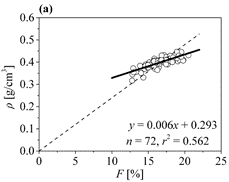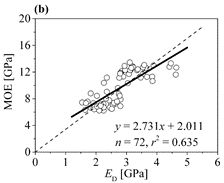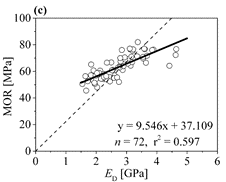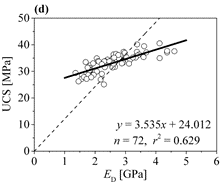Fig. 4. Relationships between nondestructive parameters and mechanical properties of small specimens: (a) ρ based on F; (b) MOE based on ED; (c) MOR based on ED; (d) UCS based on ED

The relationship between ρ and F of wood specimens is presented in Fig. 4(a). The developed regression formula between the ρ and F of wood specimens was significant at the 0.001 confidence level, with a determination of coefficient 0.562.

The regression results implied that the green density of wood specimens could be predicted by the resistance amplitude based on the resistograph test method. Rinn et al. (1996) reported that there was a significant correlation between the X-ray density profile and the gross density of dry wood obtained by the resistograph test. Some research (Rinn et al. 1996; Acuna et al. 2011; Kahl et al. 2009) also reported a high correlation between drilling resistance and density.

The relationships between ED and MOE, and MOR are presented in Fig. 4(b), (c), with determination of coefficients 0.635 and 0.597, respectively. The findings of Cavalli and Togni (2013) showed that the MOE of old beams could be predicted by using the ED, with determination of coefficients r2=0.56～0.82. The developed regression formula between them was significant at the 0.001 confidence level, which indicated that the dynamic modulus of elasticity can provide good prediction of the MOE and MOR of small specimens.

The linear relationship between ED and UCS of wood specimens is presented in Fig. 4(d), with a determination of coefficient 0.629. As reported by Zhang et al. (2015) and Calderoni et al. (2010), there are good linear relationships between the UCS and resistance amplitude in longitudinal direction, with reported coefficients of determination of r2=0.693 and 0.581, respectively. The developed regression formula between them was significant at the 0.001 confidence level, which indicated that the UCS can be predicted by the regression model.

From this study, it was known that not only the MOE but also MOR and UCS of small wood specimens can be predicted well by its dynamic modulus of elasticity. Therefore, it was suggested that the combined NDTs with resistograph and stress wave test can be effective to evaluate mechanical properties of wood specimens.

Probability Distribution

Histograms of the predicted MOR and UCS based on the nondestructive tests results are presented in Fig. 5. The probability distribution of the predicted MOR and UCS are assumed to be a normal distribution N (μ, σ2), and the cumulative distribution functions of them are as follows,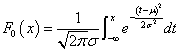(6)

where x is the random variable of the MOR and UCS predicted by the dynamic modulus of elasticity (ED). The unknown numbers μ and σ are obtained by the maximum likelihood method used in the following analysis (Table 4).

(a)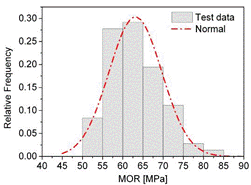(b)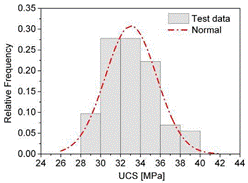Fig. 5. Normal fit the predicted MOR and UCS: (a) MOR predicted by ED; (b) UCS predicted by ED

The μ and σ values can be calculated by the maximum likelihood method as follows: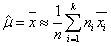(7)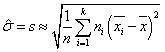(8)

where k is the sum of interval numbers, n is the total number of samples equal to the sum of ni, ni is the observed number located in the ith interval, and is the value of the interval center. The estimated μ and σ were 63.1 and 6.54 MPa for MOR, and 33.1 and 2.58 MPa for UCS, respectively.

By substitution of Eq. 7 and Eq. 8 into Eq. 6, F0(x) can be rewritten as: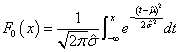(9)

Table 4. Calculation Table of,, andFurthermore, F0(x) can be transformed into a standardized normal distribution Φ(u) when u=(xμ)/σ. The cumulative distribution probability and the predicted numbers of random variable on interval [xi, xi-1] are (), and, respectively (Table 4).

F(x) is the cumulative distribution function of the predicted MOR and UCS based on the nondestructive test. Whether or not the F(x) is equal to the assumed F0(x) at the level of 0.05, it is analyzed by χ2 testing (Mao et al. 2006). The formula for χ2 is as follows: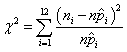(10)

Based on the Eq. 10, the calculated χ2-values of the predicted MOR and UCS were 3.61 and 4.71, respectively. For a probability level of 0.05, the critical χ2-value of the predicted MOR and UCS were 9.49, 7.81, respectively, which were less than the calculated χ2-values. Therefore, the normal distribution was judged to be a good model for the actual distribution of the predicted MOR and UCS.

Design Value of Wood Material

Design value represents the quantity of wood material, and is an important strength index that can be used to calculate the bearing capacity of structural member combined with defects of itself, such as hole, decay, etc. Therefore, the design value of wood material should be firstly determined before the calculation of bearing capacity of structural member.

According to the Chinese national standard of timber structure (GB 50005 2003), the design value of MOR and UCS of old wood were determined. The test results (Table 2) were firstly needed to transfer to the resistance. Due to the obtained test results based on the small wood specimen rather than the full size wood specimen, the bending and compressive resistance (R) of wood would be multiplied by some adjusting factors, such as the effects of the equation precision (k1), the geometric character (k2), the long-term load (k3), the natural defect (k4) (such as knot, crack and oblique grain, the drying defects (k5), the dimensions (k6) and the predicted precision of nondestructive method (k7). Therefore, the bending and compressive resistance (R) could be calculated by Eq. 11. And the mean value (µR) and coefficient of variance (δR) of R were expressed as follows (Wang 2002; Zhong et al. 2014; Zhong and Ren 2014),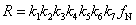(11)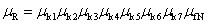(12)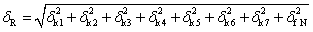(13)

where fN is the predicted value of MOR and UCS based on the nondestructive test (Table 3), with its mean value and COV shown in Table 5. And the statistical parameters of adjusting factors are shown in Table 6.

Table 5. Statistical Parameters of fN and R (GB 50005 2003)Table 6. Statistical Parameters of Adjusting Factors (GB 50005 2003)There are two types of load applied to the timber structure. One is the dead loads (G), which include the self-weight of structural members and other materials. The other is the live loads (L), which include the office occupancy load (LO), residential occupancy load (LR), wind load (LW), and snow load (LS). According to the Chinese national standard GB 50009 (2012), the statistical parameters and the distribution models of the loads are presented in Table 7.

Table 7. Statistical Parameters of the Loads (GB 50009 2012)Equation 14 is the limit state design equation for bending and compressive resistance. In determining the design value of MOR and UCS, two load combinations were used, including G+LO, G+LR, G+LW, and G+LS, respectively,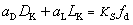(14)

where aD is the dead load factor and is equal to 1.2; aL is the live load factor and is equal to 1.4; DK is the nominal dead load; LK is the nominal live load; KS is an adjusting factor for the service life and keeps to 1.0 for 50 years; and fd is the design value of MOR and UCS predicted by nondestructive test methods (GB 50009 2012).

To calculate the design value of MOR and UCS of old wood, the performance function need to be developed for reliability analysis and could be express as (Kimiaeifar et al. 2013; Zhong et al. 2014; Zhong and Ren 2014),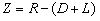(15)

where R, D, and L are random variables representing the resistance, dead load (G), and live load (LO, LR, LW, or LS), respectively. The random variable R was presumed to be a log-normal distribution (GB 50005 2003).

By substitution of Eq. 14 into Eq. 15, the performance function was rewritten as,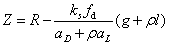(16)

where ρ, g, and l are the equivalents of LK/DK, D/DK, and L/LK, respectively.

A calculation program for reliability index (β) was developed to determine the design value of MOR and UCS based on Matlab 7 software (MathWorks, Natick, USA). First-order second-moment reliability analyses were performed for all data cells and simulation load cases, including G+LO, G+LR, G+LW, and G+LS.

For all the simulation load cases, the reliability analysis results indicated that the reliability index (β) increases nonlinearly with the increase of the live-to-dead load ratio (ρ). This is consistent with the findings of Zhuang (2004) and Li (2011). For example, the relationship between β and fd under dead load (G) plus live office load (LO), is shown in Fig. 6.

(a)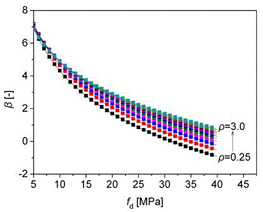(b)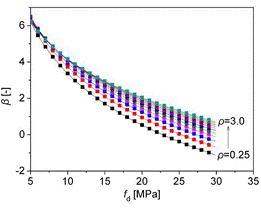Fig. 6. Relationship between reliability index (β) and design value of strength (fd) under G+LO: (a) MOR; (b) UCS

According to the Chinese national standard GB 50068 (2001), the reliability level needs to reach the target reliability level (β0=3.2) in the determination of design value of MOR and UCS, which is calculated by taking the average of the reliability index under the combinations of two loads under ρ=0.25, 0.5, 0.75, 1.0, 1.25, 1.5, 1.75, 2.0, 2.25, 2.5, 2.75, and 3.0, as shown in Fig. 7.

(a)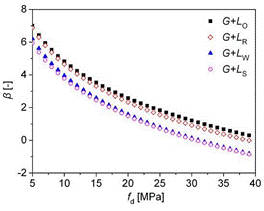(b)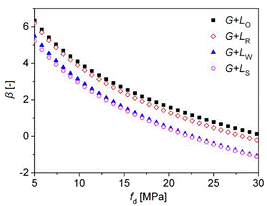Fig. 7. Relationship between reliability index (β) and design value of strength (fd) under different load combinations: (a) MOR; (b) UCS

As presented in Fig. 7, the reliability index (β) decreases nonlinearly with the increase of the design values (fd) for all the simulation load cases, including G+LO, G+LR, G+LW, and G+LS. At the same ratio of live-to-dead load (ρ) the simulation load case of the maximum and minimum β are G+LO and G+LS, respectively. Similar results have been obtained in previous studies (Zhuang 2004; Li 2011). The final reliability index obtained by taking the average of all simulation cases, is shown in Fig. 8.

According to the Chinese national standard GB 50068 (2001), a final reliability index satisfying the condition ββ0 = 3.2 is safe for engineering design. Therefore, in order to meet the target reliability level (β0 = 3.2), it is suggested that the design value of MOR and UCS be 14.0 and 10.7 MPa, respectively. The design value of ancient wood determined by nondestructive test methods, can provide a basis for calculate the bearing capacity of structural member which should contain the effects of size, defects, etc. Yang et al. (2015) reported that the coefficients of determination r2 between the dynamic MOE and static bending MOE and MOR decreased with increasing specimen sizes. A further study on the calculation the bearing capacity of structural member will be explored.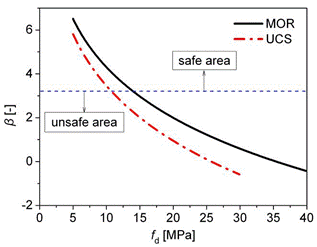Fig. 8. Prediction of the design values (fd) of strength by the reliability index (β)

CONCLUSIONS

1. The ρ, MOE, MOR, and UCS of small wood specimens could be good predictions based on the nondestructive method by stress wave timing combined with resistance drilling tests.
2. The cumulative distribution of MOR and UCS, which were predicted based on the nondestructive tests, obeyed normal distributions.
3. For all the simulation load cases, the reliability index (β) increased nonlinearly with the increase of the live-to-dead load ratio (ρ), and they decreased nonlinearly with the increase of the design values (fd).
4. According to reliability analysis, the design value of MOR and UCS of small wood specimens were determined and were 14.0 MPa and 10.7 MPa, respectively.

ACKNOWLEDGMENTS

The authors are grateful for the financial support of the National Natural Science Foundation of China (31570711) and the Grant for the Palace Museum of China (KT2015-06).

REFERENCES CITED

Acuna, L., Basterra, L.A., Casado, M., Lopez, G., Ramon-Cueto, G., Relea, E., Martinez, C., and Gonzalez, A. (2011). “Application of resistograph to obtain the density and to differentiate wood species,” Materials and Structures 61(303), 451-464. DOI: 10.3989/mc.2010.57610.

Bertolini, C., Brunetti, M., Cavallaro, P., and Macchionni, N. (1998). “A non-destructive diagnostic method on ancient timber structures: Some practical application examples,” Proceedings of 5th World Conference on Timber Engineering, Montreux, Switzerland, pp. 456-465.

Calderoni, C., De Matteis, G., Giubileo, C., and Mazzolani, F. M. (2010). “Experimental correlations between destructive and non-destructive tests on ancient timber elements,” Engineering Structures 32(2), 442-448. DOI: 10.1016/j.engstruct.2009.10.006.

Cavalli, A., and Togni, M. (2013). “How to improve the on-site MOE assessment of old timber beams combining NDT and visual strength grading,” Nondestructive Testing and Evaluation 28(3), 252-262. DOI: 10.1080/10589759.2013.764424.

Dackermann, U., Crews, K., Kasal, B., Li, J., Riggio, M., Rinn, F., and Tannert, T. (2014). “In-situ assessment of structural timber using stress-wave measurements,” Materials and Structures 47(5), 787-803. DOI: 10.1617/s11527-013-0095-4.

Feio, A., and Machado, J. S. (2015). “In-situ assessment of timber structural members: Combining information from visual strength grading and NDT/SDT methods – A review,” Construction & Building Materials 101, 1157-1165. DOI: 10.1016/j.conbuildmat.2015.05.123

GB 1936.2-2009 (2009). “Method for determination of the modulus of elasticity in static bending of wood,” Standardization Administration of China, Beijing, China.

GB 1936.1-2009 (2009). “Method of testing in bending strength of wood,” Standardization Administration of China, Beijing, China.

GB 50329-2012 (2012). “Standard for methods testing of timber structures,” Standardization Administration of China, Beijing, China.

GB 1933-2009 (2009). “Method for determination of the density of wood,” Standardization Administration of China, Beijing, China.

GB 1935-2009 (2009). “Method of testing in compressive strength parallel to grain of wood,” Standardization Administration of China, Beijing, China.

GB 50005-2003 (2003). “Code for design of timber structures,” Ministry of Housing and Urban-Rural Construction of the People’s Republic of China, China Architecture and Building Press, Beijing.

GB 50068-2001 (2001). “Unified standard for reliability design of building structures,” Ministry of Housing and Urban-Rural Construction of the People’s Republic of China, China Architecture and Building Press, Beijing.

GB 50009-2012 (2012). “Load code for the design of building structures,” Ministry of Housing and Urban-Rural Construction of the People’s Republic of China, China Architecture and Building Press, Beijing.

Kimiaeifar, A., Lund, E., Thomsen, O. T., and Sørensen, J. D. (2013). “Asymptotic sampling for reliability analysis of adhesive bonded stepped lap composite joints,” Engineering Structures 49, 655-663. DOI: 10.1016/j.engstruct.2012.12.003

Jasieńko, J., Nowak, T., and Hamrol, K. (2013). “Selected methods of diagnosis of historic timber structures—Principles and possibilities of assessment,” Advanced Materials Research 778, 225-232. DOI: 10.4028/www.scientific.net/AMR.778.225.

Kahl, T., Wirth, C., Mun, M., Böhnisch, G., and Schulze, E. (2009). “Using drill resistance to quantify the density in coarse woody debris of Norway spruce,” European Journal of Forest Research 128(5), 467-473. DOI: 10.1007/s10342-009-0294-2.

Li, T. E. (2011). Determining the Strength Design Values of Wood Based on the Reliability Requirements, Ph.D. dissertation, Harbin Institute of Technology, Harbin, China.

Mao, S. L., Wang, J. L., and Pu, X. L. (2006). Advanced Mathematical Statistics, Higher Education Press, Beijing, China.

Probett, P. S. E., Craig, C. S., and Probett, B. J. (2013). “In-situ structural timber strength measurement advances using qualitative resistography and quantitive resisto-fractometry,” in: 18th International Nondestructive Testing and Evaluation of Wood Symposium – Madison, WI.

Riggio, M., Anthony, R. W., Augelli, F., Kasal, B., Lechner, T., Muller, W., and Tannert, T. (2014). “In situ assessment of structural timber using non-destructive techniques,” Materials and Structures 47(5), 749-766. DOI: 10.1617/s11527-013-0093-6.

Rinn, F., Schweingruber, F. H., and Schär, E. (1996). “Resistograph and X-ray density charts of wood comparative evaluation of drill resistance profiles and X-ray density charts of different wood species,” Holzforschung 50(4), 303-311. DOI: 10.1515/hfsg.1996.50.4.303.

Tannert, T., Anthony, R., Kasal, B., Kloiber, M., Piazza, M., Riggio, M., Rinn, F., Widmann, R., and Yamaguchi, N. (2014). “In situ assessment of structural timber using semi-destructive techniques,” Materials and Structures 47(5), 767-785.

Tampone, G. (2001). “Acquaintance of the ancient timber structures,” in: P. B. Lourenço and P. Roca (eds.), Historical Constructions, Guimarães.

Wang, X., Divos, F., Pilon, C., Brashaw, B. K., Ross, R. J., and Pellerin, R. F. (2004). Assessment of Decay in Standing Timber Using Stress Wave Timing Nondestructive Evaluation Tools (Technical Report FPLGTR-147), U. S. Department of Agriculture Forest Products Laboratory, Madison, WI, USA.

Wang, Y. W. (2002). “Reliability analysis on timber structure,” Building Science Research of Sichuan 28(2), 1-2, 9. DOI: 10.3969/j.issn.1008-1933.2002.02.001

Yang, B. Z., Seale, R. D., Shmulsky, R., and Wang, X. (2015). “Comparison of nondestructive testing methods for evaluating No.2 southern pine lumber: Part A, Modulus of elasticity,” Wood & Fiber Science 47(4), 375-384.

Yin, Y. F., Nagao, H., Liu, X. L., and Nakai, T. (2010). “Mechanical properties assessment of Cunninghamia lanceolata plantation wood with three acoustic-based nondestructive methods,” Journal of Wood Science 56, 33-40. DOI: 10.1007/s10086-009-1067-8.

Zhang, J., Xu, Q. F., Xu, Y. X., and Zhang, M. (2015). “Research on residual bending capacities of used wood members based on the correlation between non-destructive testing results and the mechanical properties of wood,” Journal of Zhejiang University-SCIENCE A (Applied Physics & Engineering) 16(7), 541-550. DOI: 10.1631/jzus.A1400276

Zhong, Y., Jiang, Z. H., Shangguan, W. W., and Ren, H. Q. (2014). “Design value of the compressive strength for bamboo fiber-reinforced composite based on a reliability analysis,” BioResources 9(4), 7737-7748. DOI: 10.15376/biores.9.4.7737-774810

Zhong, Y., and Ren, H. Q. (2014). “Reliability analysis for the bending strength of larch 2 × 4 lumber,” BioResources 9(4), 6914-6923. DOI: 10.15376/biores.9.4.6914-6923

Zhuang, X. J. (2004). Reliability Study of North American Dimension Lumber in the Chinese Timber Structures Design Code, Ph.D. Dissertation, University of British Columbia, Vancouver, BC.

Article submitted: September 7, 2016; Peer review completed: November 20, 2016; Revised version received: January 16, 2017; Published: February 6, 2017.

DOI: 10.15376/biores.12.2.2310-2325